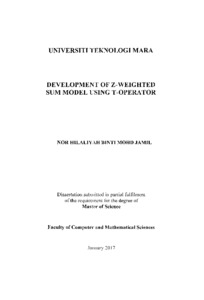# Development of Z-weighted sum model using T-operator / Nor Hilaliyah Mohd Jamil

Mohd Jamil, Nor Hilaliyah (2017) Development of Z-weighted sum model using T-operator / Nor Hilaliyah Mohd Jamil. Masters thesis, Universiti Teknologi MARA.

## Abstract

Z-number is a concept that has been receiving more attention in recent years. The increment of Z-number usage in decision making problem recently, has given huge impact in the research world especially under fuzzy environment. In order to measure for the uncertainty, the reliability factors are considered in the information evaluation. A Z-number is an ordered pair of fuzzy numbers, Z= (A, R) which consist of restriction, A and reliability, R. In Z-number, the reliability can be described as the concept of confidence level, sureness or the probability that consists a value between zero and one. Unlike fuzzy number, Z-number has more ability to explain the uncertain information and measure the reliability of the information more accurately. However, the calculation of the reliability in Z-number is still not consistent. The existing method introduce by the researcher, may not portray the reliability's value specifically, where it leads to loss of information through the calculation process. Thus, a new proposed method is introduced to cater this problem. The aims of this study are to develop an arithmetic operation, which involves with addition and multiplication operation based on the t-operator that are able to solve for the reliability in Z-number. These operations are suitable to be used due to the t-operator has the same behaviour with the probability concept where it used the value between zero and one. In this dissertation, there are three proposed arithmetic operations based on Tofan (2013) that use different types of t-operator, which are Einstein and Hamacher and combination of both. The proving of the proposed arithmetic operations are shown to prove that these operations are valid to be used. Then, the concept of Z-number is implemented in one of the Multi Criteria Decision Making methods (MCDM), which is a Weighted Sum Model (WSM) which later known as Z-WSM. The proposed arithmetic operations are used to aggregate the weighted of criteria and the rating of alternatives in the calculation of reliability in ZWSM. Later, a comparative analysis is conducted to compare for the value of relative closeness and their ranking between the proposed methods and Tofan based on numerical example from paper Chen (2000). An analysis of each proposed arithmetic operation of Z-WSM methods has been presented. The results show that each of the methods share the same ranking value where candidates 2, E₂ was chosen as the best candidates. Finally, the Z-WSM is applied in the case study of store location selection and the result shows that among the four different locations, Kota Damansara is chosen as the preferred location for opening new stores. These proposed arithmetic operations can be used as an alternative way in solving Z-number where the reliability information can be preserved and the value of probability in the reliability can be presented through the calculation process.

Item Type: Thesis (Masters)
Creators:
CreatorsID Num. / Email
Mohd Jamil, Nor HilaliyahUNSPECIFIED
Subjects: Q Science > QA Mathematics > Instruments and machines > Electronic computers. Computer science
Q Science > QA Mathematics > Instruments and machines > Electronic computers. Computer science
Divisions: Universiti Teknologi MARA, Shah Alam > Faculty Computer and Mathematical Sciences
Item ID: 21577
Uncontrolled Keywords: Z-weighted sum model; T-operator
URI: http://ir.uitm.edu.my/id/eprint/21577Preview
Text
TM_NOR HILALIYAH MOHD JAMIL CS 17_5.pdf

## Fulltext

Fulltext is available at:
UNSPECIFIED

## ID Number

### 21577View Item